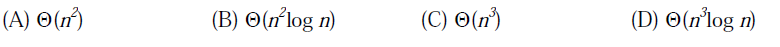# GATE | GATE CS 2013 | Question 19

What is the time complexity of Bellman-Ford single-source shortest path algorithm on a complete graph of n vertices?(A) A
(B) B
(C) C
(D) D

Explanation: Time complexity of Bellman-Ford algorithm is O(VE) where V is number of vertices and E is number of edges. For a complete graph with n vertices, V = n, E = O(n^2). So overall time complexity becomes O(n^3)

Quiz of this Question

My Personal Notes arrow_drop_up
Article Tags :

1

Please write to us at contribute@geeksforgeeks.org to report any issue with the above content.